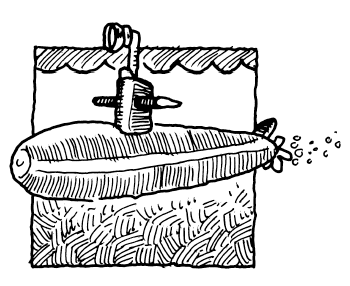### Home > PC > Chapter 9 > Lesson 9.2.2 > Problem9-84

9-84.Two submarines left the Long Beach Shipyard, one at a speed of $25$ knots and the other at a speed of $35$ knots. Their courses diverged by $35^\circ$. After $3$ hours, how far apart are they? (Note: A knot is a measure of speed defined as one nautical mile per hour. A nautical mile is $1852$ meters or $1.852$ kilometers.)

Draw and label a diagram. Note that the values given are speeds, not distances.

Once you have calculated the distances the submarines have each traveled after $3$ hours, use the Law of Cosines.

$c^2=a^2+b^2−2ab\cos\left(C\right)$